The vector fields developed in the last section yield feasible trajectories, but not necessarily optimal trajectories unless the initial and goal states are in the same convex-cell. If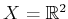, then it is possible to make a continuous version of Dijkstra's algorithm . This results in an exact cost-to-go function overbased on the Euclidean shortest path to a goal,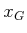. The cost-to-go function serves as the navigation function, from which the feedback plan is defined by using a local steepest descent.

Suppose thatis bounded by a simple polygon (no holes). Assume that only normalized vector fields are allowed. The cost functional is assumed to be the Euclidean distance traveled along a state trajectory. Recall from Section 6.2.4 that for optimal path planning,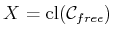must be used. Assume thatand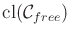have the same connectivity.8.12 This technically interferes with the definition of tangent spaces from Section 8.3 because each point ofmust be contained in an open neighborhood. Nevertheless, we allow vectors along the boundary, provided that they point'' in a direction tangent to the boundary. This can be formally defined by considering boundary regions as separate manifolds.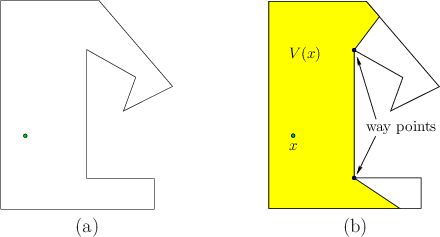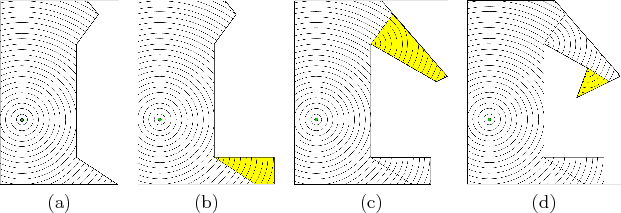Consider computing the optimal cost-to-go to a pointfor a problem such as that shown in Figure 8.12a. For any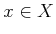, let the visibility polygon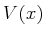refer to the set of all points visible from, which is illustrated in Figure 8.12b. A point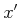lies inif and only if the line segment fromtois contained in. This implies that the cost-to-go fromtois just the Euclidean distance fromto. The optimal navigation function can therefore be immediately defined over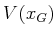as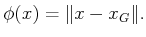(8.42)

Level sets at regularly spaced values of this navigation function are shown in Figure 8.13a.

How do we compute the optimal cost-to-go values for the points in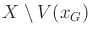? For the segments on the boundary offor any, some edges are contained in the boundary of, and others cross the interior of. For the example in Figure 8.12b, there are two edges that cross the interior. For each segment that crosses the interior, let the closer of the two vertices tobe referred to as a way point. Two way points are indicated in Figure 8.12b. The way points ofare places through which some optimal paths must cross. Let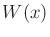for anydenote the set of way points of.

A straightforward algorithm proceeds as follows. Let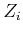denote the set of points over which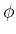has been defined, in theth iteration of the algorithm. In the first iteration,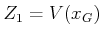, which is the case shown in Figure 8.13a. The way points ofare placed in a queue,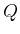. In each following iteration, a way pointis removed from. Letdenote the domain over whichis defined so far. The domain ofis extended to include all new points visible from. These new points are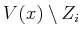. This yields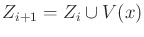, the extended domain of. The values of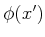for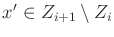are defined by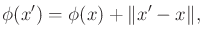(8.43)

in whichis the way point that was removed from(the optimal cost-to-go value ofwas already computed). The way points ofthat do not lie in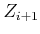are added to. Each of these will yield new portions ofthat have not yet been seen. The algorithm terminates whenis empty, which implies that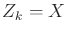for some. The execution of the algorithm is illustrated in Figure 8.13.

The visibility polygon can be computed in time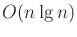ifis described byedges. This is accomplished by performing a radial sweep, which is an adaptation of the method applied in Section 6.2.2 for vertical cell decomposition. The difference for computingis that a ray anchored atis swept radially (like a radar sweep). The segments that intersect the ray are sorted by their distance from. For the algorithm that constructs the navigation function, no more than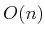visibility polygons are computed because each one is computed from a unique way point. This implies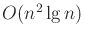running time for the whole algorithm. Unfortunately, there is no extension to higher dimensions; recall from Section 7.7.1 that computing shortest paths in a 3D environment is NP-hard .

The algorithm given here is easy to describe, but it is not the most general, nor the most efficient. Ifhas holes, then the level set curves can collide by arriving from different directions when traveling around an obstacle. The queue,, described above can be sorted as in Dijkstra's algorithm, and special data structures are needed to identify when critical events occur as the cost-to-go is propagated outward. It was shown in  that this can be done in timeand space.

Steven M LaValle 2020-08-14